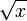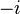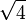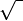# Square root facts for kids

Kids Encyclopedia Facts

In mathematics, a square root of a number x is another number that, when multiplied by itself (squared), becomes x. When it is non-negative, it is represented by the symbol$\sqrt{x}$, and called the principal square root of x. For example, 2 is the square root of 4, because 2×2=4. Only numbers bigger than or equal to zero have real square roots. The only square root of zero is zero.

An important number is the square root of 2, which is an irrational number. Its value is around 1.41421. It is also the length of a diagonal in the square whose side is 1.

Square roots of negative numbers are not real numbers – they are imaginary numbers. Imaginary numbers are basically numbers whose square is negative. Every complex number, except 0, has 2 square roots. For example, −1 has two square roots. We call them$i$ and$-i$. We also consider the number$i$ as the principal square root of −1, and called it the imaginary unit.

The sign for a square root is made by putting a bent line over a number, like this:$\sqrt 4$. This is read as "the square root of 4" (or whatever number we are taking the square root of).

A whole number with a square root that is also a whole number is called a perfect square. The first few perfect squares are: 0, 1, 4, 9, 16, 25, 36, 49, 64, 81, 100, 121, 144, 169, 196, 225, 256, 289, 324, 361, 400, 441, 484, 529, 576, 625, 676, 729, 784, 841, 900, 961, 1024, 1089, 1156, 1225.

Below is a table of square roots (rounded to 3 decimals places).

Number Square root of number
1 1.000
2 1.414
3 1.732
4 2.000
5 2.236
6 2.449
7 2.646
8 2.828
9 3.000
10 3.162
11 3.317
12 3.464
13 3.606
14 3.742
15 3.873
16 4.000
17 4.123
18 4.243
19 4.359
20 4.472

## Origin of the symbol

It is not really known where the square root symbol$\sqrt{\,\,}$ comes from, but some people believe that it was from the letter r, which is the first letter of the Latin and German word radix. Radix means root or base. Thus, radix quadratum from Latin refer most likely to the base of a square. Since the sides of a square are all equal, the word radix may be interpreted as side of a square—without actually so.

## Images for kidsIn Spanish: Raíz cuadrada para niños